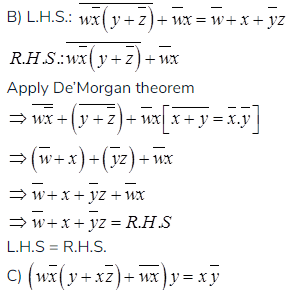# GATE Solved Paper 2017-19 - GATE 2017 Shift 2

>>>>>>>>GATE 2017 Shift 2

• A

p = 3 and q = 1• B

p = 3 and q = 2• C

p = 4 and q = 1• D

p = 4 and q = 2• Option : A
• Explanation :
Given:
code1 00000
code2 01011
code3 10101
code4 11110
Hamming distance between code 1 and code 2 is 3
Hamming distance between code 1 and code 3 is 3
Hamming distance between code 1 and code 4 is 4
Hamming distance between code 2 and code 3 is 4
Hamming distance between code 2 and code 4 is 3
Hamming distance between code 3 and code 4 is 3
So, as per Hamming code, minimum Hamming distance of all code words is considered as Hamming distance i.e., 3 (p).
Now, the max number of erroneous bits that can be corrected by the Hamming code is 2d + 1
So,
So option A is correct.

Which of the following best describes current state of the system ?

• A• B• C• D• Option : B
• Explanation :  PID Current allocation Max Need Available Needed P1 3 3 2 0 P2 1 6 - 5 P3 3 5 - 2
Hence, system is in safe state , because P1 and P3 anyone can execute first and after them P2 can be executed, no deadlocked option B is correct.

• A

54• B

55• C

56• D

57• Option : A
• Explanation :
Conflict conditions RW WR WW
∴ 5 conflicts
T1 - T2
a  b  c  d
r1(X) w1(X) r1(Y) w1(Y)
r2(Y) w2(Y) r2(Z) r2(Z)
1  2  3  4
Constraints:
a<b<c<d
1<2<3<4
d<1 (or) 2<c
only 1 way
Total = 70 - (12 + 5) = 53
Therefore total no of, 53 + 1 = 54

• Option : C
• Explanation :
A)LHS: wx + w(x + y) + x(x+y) = x + wy
RHS
⇒ wx + wx + wy + x + xy
⇒ wx + wy + x + xy [∵ xx = x]
⇒ x(w+y+1) + wy [∵1 + x = 1]
⇒ x + wy
⇒ L.H.S = R.H.SL.H.S ≠ R.H.S
D) L.H.S.:(w + y)(wxy + wyz) = wxy + wyz
(w + y)(wxy + wyz)
wxy + wyz + wxy + wyz
wxy + wyz
L.H.S = R.H.S

Note – Numerical Type question

• A

2• B

4• C

6• D

8• Option : A
• Explanation :
Assume starting address of string is 100 to make the whole expression is easy to understand.Note: Whenever we have characters in the arithmetic expressions, we can replace those with their ASCII values
strlen (100 + x + 11 - x - 1)[assume x has the ASCII value of I]
⇒Strlen(110)
is printed
It gives address of second last character in the string. So it prints length 2.
Related Quiz.
GATE 2017 Shift 2